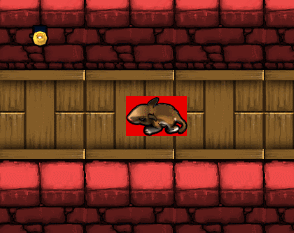Contact Home

# Auto calculating bounding box from texture in Monogame / XNA

My current project involves thousands of frames of animation. If I were to determine the bounding boxes of all these frames by hand, it would be very tedious. Instead, I decided to auto calculate all the bounding boxes. To better illustrate this, take a look at the following animation:Notice how the red portion (bounding box) automatically re-sizes based on the current frame of the animation. Having these dynamic bounding boxes helps greatly when dealing with collision detection and collision response.

The idea behind this is quite simple. When loading your images into your game, you calculate the smallest rectangle possible, based on the pixel data. If a pixel is transparent, we do not include it in our rectangle, otherwise, we do. Take a look at the following code:
```public static class CalculateBounds
{
//Get smallest rectangle from Texture, cased on color
public static Rectangle GetSmallestRectangleFromTexture(Texture2D Texture)
{
//Create our index of sprite frames
Color[,] Colors = TextureTo2DArray(Texture);

//determine the min/max bounds
int x1 = 9999999, y1 = 9999999;
int x2 = -999999, y2 = -999999;

for (int a = 0; a < Texture.Width; a++)
{
for (int b = 0; b < Texture.Height; b++)
{
//If we find a non transparent pixel, update bounds if required
if (Colors[a, b].A != 0)
{
if (x1 > a) x1 = a;
if (x2 < a) x2 = a;

if (y1 > b) y1 = b;
if (y2 < b) y2 = b;
}
}
}

//We now have our smallest possible rectangle for this texture
return new Rectangle(x1, y1, x2 - x1 + 1, y2 - y1 + 1);
}

//convert texture to 2d array
private static Color[,] TextureTo2DArray(Texture2D texture)
{
//Texture.GetData returns a 1D array
Color[] colors1D = new Color[texture.Width * texture.Height];
texture.GetData(colors1D);

//convert the 1D array to 2D for easier processing
Color[,] colors2D = new Color[texture.Width, texture.Height];
for (int x = 0; x < texture.Width; x++)
for (int y = 0; y < texture.Height; y++)
colors2D[x, y] = colors1D[x + y * texture.Width];

return colors2D;
}
}
```

You can further optimize by running the calculations once, and saving the bounds data to file. Then load the pre-calculated data when you run your game.

Contact me with any questions or comments.

# 2 Responses

## Krishancommented on September 29, 2017 at 5:51 amHey there,

First of all thanks for this great article!

I would like to know how you calculate the position of the bounding box? Our maybe I should ask more about how you calculate your characters position if the texture is higher/wider than the character itself?

Would love to read more monogame tutorials by you.

Best regards,
Krishan